Home
Hostname: page-component-55597f9d44-ms7nj Total loading time: 0.471 Render date: 2022-08-15T04:32:24.316Z Has data issue: true Feature Flags: { "shouldUseShareProductTool": true, "shouldUseHypothesis": true, "isUnsiloEnabled": true, "useRatesEcommerce": false, "useNewApi": true } hasContentIssue true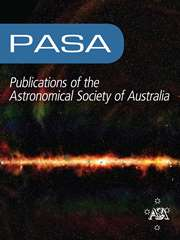Publications of the Astronomical Society of Australia

# The GLEAM 4-Jy (G4Jy) Sample: II. Host galaxy identification for individual sources

Published online by Cambridge University Press:  01 June 2020

*
Author for correspondence: Sarah V. White, E-mail: sarahwhite.astro@gmail.com

## Abstract

The entire southern sky (Declination,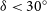$\delta< 30^{\circ}$ ) has been observed using the Murchison Widefield Array (MWA), which provides radio imaging of$\sim$ 2 arcmin resolution at low frequencies (72–231 MHz). This is the GaLactic and Extragalactic All-sky MWA (GLEAM) Survey, and we have previously used a combination of visual inspection, cross-checks against the literature, and internal matching to identify the ‘brightest’ radio-sources (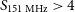$S_{\mathrm{151\,MHz}}>4$ Jy) in the extragalactic catalogue (Galactic latitude,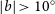$|b| >10^{\circ}$ ). We refer to these 1 863 sources as the GLEAM 4-Jy (G4Jy) Sample, and use radio images (of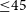${\leq}45$ arcsec resolution), and multi-wavelength information, to assess their morphology and identify the galaxy that is hosting the radio emission (where appropriate). Details of how to access all of the overlays used for this work are available at https://github.com/svw26/G4Jy. Alongside this we conduct further checks against the literature, which we document here for individual sources. Whilst the vast majority of the G4Jy Sample are active galactic nuclei with powerful radio-jets, we highlight that it also contains a nebula, two nearby, star-forming galaxies, a cluster relic, and a cluster halo. There are also three extended sources for which we are unable to infer the mechanism that gives rise to the low-frequency emission. In the G4Jy catalogue we provide mid-infrared identifications for 86% of the sources, and flag the remainder as: having an uncertain identification (129 sources), having a faint/uncharacterised mid-infrared host (126 sources), or it being inappropriate to specify a host (2 sources). For the subset of 129 sources, there is ambiguity concerning candidate host-galaxies, and this includes four sources (B0424–728, B0703–451, 3C 198, and 3C 403.1) where we question the existing identification.

Type
Research Article

## Access options

Get access to the full version of this content by using one of the access options below. (Log in options will check for institutional or personal access. Content may require purchase if you do not have access.)

## References

Abell, G. O., Corwin, H. G. Jr, & Olowin, R. P. 1989, ApJS, 70, 1CrossRefGoogle Scholar
Alam, S., et al. 2015, ApJS, 219, 12CrossRefGoogle Scholar
Aniyan, A. K., & Thorat, K. 2017, ApJS, 230, 20CrossRefGoogle Scholar
Antonucci, R. R. J. 1985, ApJS, 59, 499CrossRefGoogle Scholar
Astropy Collaboration, et al. 2013, A&A, 558, A33Google Scholar
Bagchi, J., Pislar, V., & Lima Neto, G. B. 1998, MNRAS, 296, L23Google Scholar
Banfield, J. K., et al. 2015, MNRAS, 453, 2326CrossRefGoogle Scholar
Baum, S. A., Heckman, T. M., Bridle, A., van Breugel, W. J. M., & Miley, G. K. 1988, ApJS, 68, 643CrossRefGoogle Scholar
Baum, S. A., et al. 1997, ApJ, 483, 178CrossRefGoogle Scholar
Bennett, A. S. 1962, MNRAS, 125, 75CrossRefGoogle Scholar
Best, P. N., Longair, M. S., & Roettgering, H. J. A. 1997, MNRAS, 292, 758CrossRefGoogle Scholar
Bicknell, G. V., Cameron, R. A., & Gingold, R. A. 1990, ApJ, 357, 373CrossRefGoogle Scholar
Bik, A., Lenorzer, A., Kaper, L., Comerón, F., Waters, L. B. F. M., de Koter, A., & Hanson, M. M. 2003, A&A, 404, 249Google Scholar
Black, A. R. S., Baum, S. A., Leahy, J. P., Perley, R. A., Riley, J. M., & Scheuer, P. A. G. 1992, MNRAS, 256, 186CrossRefGoogle Scholar
Blandford, R. D., & Königl, A. 1979, ApJ, 232, 34CrossRefGoogle Scholar
Blandford, R. D., & Payne, D. G. 1982, MNRAS, 199, 883CrossRefGoogle Scholar
Blandford, R. D., & Znajek, R. L. 1977, MNRAS, 179, 433CrossRefGoogle Scholar
Bolton, J. G., Clarke, M. E., & Ekers, R. D. 1965, AuJP, 18, 627Google Scholar
Bondi, M., Gregorini, L., Padrielli, L., & Parma, P. 1993, A&AS, 101, 431Google Scholar
Burgess, A. M., & Hunstead, R. W. 2006a, AJ, 131, 100CrossRefGoogle Scholar
Burgess, A. M., & Hunstead, R. W. 2006b, AJ, 131, 114CrossRefGoogle Scholar
Burns, J. O., & Owen, F. N. 1979, AJ, 84, 1478CrossRefGoogle Scholar
Buttiglione, S., Capetti, A., Celotti, A., Axon, D. J., Chiaberge, M., Macchetto, F. D., & Sparks, W. B. 2010, A&A, 509, A6Google Scholar
Capetti, A., Zamfir, S., Rossi, P., Bodo, G., Zanni, C., & Massaglia, S. 2002, A&A, 394, 39Google Scholar
Condon, J. J., Cotton, W. D., Greisen, E. W., Yin, Q. F., Perley, R. A., Taylor, G. B., & Broderick, J. J. 1998, AJ, 115, 1693CrossRefGoogle Scholar
Cox, C. I., Gull, S. F., & Scheuer, P. A. G. 1991, MNRAS, 252, 558CrossRefGoogle Scholar
Croft, S., et al. 2006, ApJ, 647, 1040CrossRefGoogle Scholar
Croston, J. H., Hardcastle, M. J., Birkinshaw, M., Worrall, D. M., & Laing, R. A. 2008, MNRAS, 386, 1709CrossRefGoogle Scholar
Croton, D. J., et al. 2006, MNRAS, 365, 11CrossRefGoogle Scholar
Cutri, R. M., et al. 2013, VizieR Online Data Catalog, 2328Google Scholar
da Cunha, E., et al. 2017, PASA, 34, e047CrossRefGoogle Scholar
de Grijp, M. H. K., Keel, W. C., Miley, G. K., Goudfrooij, P., & Lub, J. 1992, A&AS, 96, 389Google Scholar
Danziger, I. J., & Goss, W. M. 1983, MNRAS, 202, 703CrossRefGoogle Scholar
De Breuck, C., van Breugel, W., Röttgering, H. J. A., & Miley, G. 2000, A&AS, 143, 303Google Scholar
Duncan, R. A., & Sproats, L. N. 1992, PASAu, 10, 16CrossRefGoogle Scholar
Durdin, J. M., Pleticha, D., Condon, J. J., Yerbury, M. J., Jauncey, D. L., & Hazard, C. 2000, VizieR Online Data Catalog, 8061Google Scholar
Ekers, R. D., et al. 1989, MNRAS, 236, 737CrossRefGoogle Scholar
Emonts, B. H. C., et al. 2011, ApJ, 734, 25CrossRefGoogle Scholar
Ensslin, T. A., Biermann, P. L., Klein, U., & Kohle, S. 1998, A&A, 332, 395Google Scholar
Fujita, Y., Sarazin, C. L., Kempner, J. C., Rudnick, L., Slee, O. B., Roy, A. L., Andernach, H., & Ehle, M. 2002, ApJ, 575, 764CrossRefGoogle Scholar
Gaibler, V., Khochfar, S., Krause, M., & Silk, J. 2012, MNRAS, 425, 438CrossRefGoogle Scholar
Gallais, P., Rouan, D., Lacombe, F., Tiphene, D., & Vauglin, I. 1991, A&A, 249, 309Google Scholar
García-Burillo, S., et al. 2014, A&A, 567, A125Google Scholar
Giacintucci, S., Markevitch, M., Venturi, T., Clarke, T. E., Cassano, R., & Mazzotta, P. 2014, ApJ, 781, 9CrossRefGoogle Scholar
Gilbert, G. M., Riley, J. M., Hardcastle, M. J., Croston, J. H., Pooley, G. G., & Alexander, P. 2004, MNRAS, 351, 845CrossRefGoogle Scholar
Godfrey, L. E. H., et al. 2009, ApJ, 695, 707CrossRefGoogle Scholar
Goss, W. M., Ekers, R. D., Skellern, D. J., & Smith, R. M. 1982, MNRAS, 198, 259CrossRefGoogle Scholar
Govoni, F., et al. 2017, A&A, 603, A122Google Scholar
Gregorini, L., de Ruiter, H. R., Parma, P., Sadler, E. M., Vettolani, G., & Ekers, R. D. 1994, A&AS, 106, 1Google Scholar
Griffith, M. R., Wright, A. E., Burke, B. F., & Ekers, R. D. 1995, ApJS, 97, 347CrossRefGoogle Scholar
Hambly, N. C., et al. 2001, MNRAS, 326, 1279CrossRefGoogle Scholar
Hardcastle, M. J. 2018, MNRAS, 475, 2768CrossRefGoogle Scholar
Hardcastle, M. J., et al. 2019, MNRAS, 488, 3416CrossRefGoogle Scholar
Heald, G., et al. 2016, MNRAS, 462, 1238CrossRefGoogle Scholar
Hindson, L., et al. 2014, MNRAS, 445, 330CrossRefGoogle Scholar
Hovatta, T., O’Sullivan, S., Martí-Vidal, I., Savolainen, T., & Tchekhovskoy, A. 2019, A&A, 623, A111Google Scholar
Hunstead, R. W., Murdoch, H. S., Condon, J. J., & Phillips, M. M. 1984, MNRAS, 207, 55CrossRefGoogle Scholar
Hunter, J. D. 2007, Computing in Science & Engineering, 9, 90CrossRefGoogle Scholar
Hurley-Walker, N., et al. 2017, MNRAS, 646, 1146CrossRefGoogle Scholar
Intema, H. T., Jagannathan, P., Mooley, K. P., & Frail, D. A. 2017, A&A, 598, A78Google Scholar
Jackson, C. A., et al. 2015, in The Many Facets of Extragalactic Radio Surveys: Towards New Scientific Challenges. (arXiv:1604.04041)Google Scholar
Jenkins, C. J., Pooley, G. G., & Riley, J. M. 1977, MmRAS, 84, 61Google Scholar
Jetha, N. N., Hardcastle, M. J., & Sakelliou, I. 2006, MNRAS, 368, 609CrossRefGoogle Scholar
Johnston-Hollitt, M., Hunstead, R. W., & Corbett, E. 2008, A&A, 479, 1Google Scholar
Jones, P. A. 1989, PASAu, 8, 81CrossRefGoogle Scholar
Jones, P. A. 1992, AuJP, 45, 797Google Scholar
Jones, P. A., & McAdam, W. B. 1992, ApJS, 80, 137CrossRefGoogle Scholar
Jones, E., et al. 2001, SciPy: open source scientific tools for Python, http://www.scipy.org/Google Scholar
Jones, D. H., et al. 2004, MNRAS, 355, 747CrossRefGoogle Scholar
Jones, D. H., et al. 2009, MNRAS, 399, 683CrossRefGoogle Scholar
Joye, W. A., & Mandel, E. 2003, in Astronomical Data Analysis Software and Systems XII, ed. Payne, H. E., Jedrzejewski, R. I., & Hook, R. N., Astronomical Society of the Pacific Conference Series (Vol. 295), 489Google Scholar
Kapahi, V. K., Athreya, R. M., Subrahmanya, C. R., Baker, J. C., Hunstead, R. W., McCarthy, P. J., & van Breugel, W. 1998, ApJ, 118, 327Google Scholar
Kapińska, A. D., et al. 2017, ApJ, 838, 68CrossRefGoogle Scholar
Kellermann, K. I., Pauliny-Toth, I. I. K., & Williams, P. J. S. 1969, ApJ, 157, 1CrossRefGoogle Scholar
Kimball, A. E., & Ivezić, Ž. 2008, AJ, 136, 684CrossRefGoogle Scholar
Kimball, A. E., & Ivezić, Ž., 2014, in Multiwavelength AGN Surveys and Studies, ed. Mickaelian, A. M. & D. B., Sanders IAU Symposium (Vol. 304), 238. doi:10.1017/S1743921314003901CrossRefGoogle Scholar
Kuźmicz, A., Jamrozy, M., Kozieł-Wierzbowska, D., & Weżgowiec, M. 2017, MNRAS, 471, 3806CrossRefGoogle Scholar
Laing, R. A., Riley, J. M., & Longair, M. S. 1983, MNRAS, 204, 151CrossRefGoogle Scholar
Leahy, J. P., & Perley, R. A. 1991, AJ, 102, 537CrossRefGoogle Scholar
Leahy, J. P., Pooley, G. G., & Riley, J. M. 1986, MNRAS, 222, 753CrossRefGoogle Scholar
Leahy, J. P., Bridle, A. H., & Strom, R. G. 1996, in Extragalactic Radio Sources, ed. Ekers, R. D., Fanti, C., & Padrielli, L., IAU Symposium (Vol. 175), 157CrossRefGoogle Scholar
Leahy, J. P., Black, A. R. S., Dennett-Thorpe, J., Hardcastle, M. J., Komissarov, S., Perley, R. A., Riley, J. M., & Scheuer, P. A. G. 1997, MNRAS, 291, 20CrossRefGoogle Scholar
Lenc, E., & Tingay, S. J. 2006, AJ, 132, 1333CrossRefGoogle Scholar
Liuzzo, E., Giovannini, G., Giroletti, M., & Taylor, G. B. 2010, A&A, 516, A1Google Scholar
Mahony, E. K., et al. 2011, MNRAS, 417, 2657CrossRefGoogle Scholar
Malarecki, J. M., Jones, D. H., Saripalli, L., Staveley-Smith, L., & Subrahmanyan, R. 2015, MNRAS, 449, 955CrossRefGoogle Scholar
Massaro, F., et al. 2012, ApJS, 203, 31CrossRefGoogle Scholar
Mauch, T., Murphy, T., Buttery, H. J., Curran, J., Hunstead, R. W., Piestrzynski, B., Robertson, J. G., & Sadler, E. M. 2003, MNRAS, 342, 1117CrossRefGoogle Scholar
McAlpine, K., Smith, D. J. B., Jarvis, M. J., Bonfield, D. G., & Fleuren, S. 2012, MNRAS, 423, 132CrossRefGoogle Scholar
McCarthy, P. J., Kapahi, V. K., van Breugel, W., Persson, S. E., Athreya, R., & Subrahmanya, C. R. 1996, ApJS, 107, 19CrossRefGoogle Scholar
Merritt, D., & Ekers, R. D. 2002, Sci, 297, 1310CrossRefGoogle Scholar
Miley, G. K. 1973, A&A, 26, 413Google Scholar
Miley, G. 1980, ARA&A, 18, 165CrossRefGoogle Scholar
Minkowski, R. 1958, PASP, 70, 143CrossRefGoogle Scholar
Morganti, R., Killeen, N. E. B., & Tadhunter, C. N. 1993, MNRAS, 263, 1023CrossRefGoogle Scholar
Morganti, R., Oosterloo, T., Tadhunter, C. N., Aiudi, R., Jones, P., & Villar-Martin, M. 1999, A&AS, 140, 355Google Scholar
Morganti, R., Fogasy, J., Paragi, Z., Oosterloo, T., & Orienti, M. 2013, Sci, 341, 1082CrossRefGoogle Scholar
Mukherjee, D., Bicknell, G. V., Sutherland, R., & Wagner, A. 2016, MNRAS, 461, 967CrossRefGoogle Scholar
Murgia, M., Parma, P., de Ruiter, H. R., Bondi, M., Ekers, R. D., Fanti, R., & Fomalont, E. B. 2001, A&A, 380, 102Google Scholar
Murphy, T., Mauch, T., Green, A., Hunstead, R. W., Piestrzynska, B., Kels, A. P., & Sztajer, P. 2007, MNRAS, 382, 382CrossRefGoogle Scholar
Murphy, T., et al. 2010, MNRAS, 402, 2403CrossRefGoogle Scholar
Nilsson, K. 1998, A&AS, 132, 31Google Scholar
Norris, R. P., et al. 2006, AJ, 132, 2409CrossRefGoogle Scholar
O’Dea, C. P., & Owen, F. N. 1985, AJ, 90, 927CrossRefGoogle Scholar
Ochsenbein, F., Bauer, P., & Marcout, J. 2000, A&AS, 143, 23Google Scholar
Oliphant, T. 2006, Guide to NumPy (Trelgol Publishing). http://www.tramy.us/numpybook.pdfGoogle Scholar
Orenstein, B. J., Collier, J. D., & Norris, R. P. 2019, MNRAS, 484, 1021CrossRefGoogle Scholar
Owen, F. N., & Ledlow, M. J. 1997, ApJS, 108, 41CrossRefGoogle Scholar
Owen, F. N., O’Dea, C. P., Inoue, M., & Eilek, J. A. 1985, ApJ, 294, L85CrossRefGoogle Scholar
Owen, F. N., White, R. A., & Burns, J. O. 1992, ApJS, 80, 501CrossRefGoogle Scholar
Owers, M. S., Couch, W. J., & Nulsen, P. E. J. 2009, ApJ, 693, 901CrossRefGoogle Scholar
Rawlings, S., Lacy, M., Leahy, J. P., Dunlop, J. S., Garrington, S. T., & Ludke, E. 1996, MNRAS, 279, L13CrossRefGoogle Scholar
Rees, M. J. 1966, Nature, 211, 468CrossRefGoogle Scholar
Reid, A. D. 1999, PhD thesis, University of SydneyGoogle Scholar
Reid, R. I., Kronberg, P. P., & Perley, R. A. 1999, ApJS, 124, 285CrossRefGoogle Scholar
Reynolds, J. E. 1980, PASAu, 4, 74CrossRefGoogle Scholar
Rieke, G. H., Lebofsky, M. J., Thompson, R. I., Low, F. J., & Tokunaga, A. T. 1980, ApJ, 238, 24CrossRefGoogle Scholar
Riseley, C. J., Scaife, A. M. M., Oozeer, N., Magnus, L., & Wise, M. W. 2015, MNRAS, 447, 1895CrossRefGoogle Scholar
Riseley, C. J., et al. 2018, PASA, 35, 43CrossRefGoogle Scholar
Rizza, E., Loken, C., Bliton, M., Roettiger, K., Burns, J. O., & Owen, F. N. 2000, AJ, 119, 21CrossRefGoogle Scholar
Robitaille, T., & Bressert, E. 2012, APLpy: Astronomical Plotting Library in Python (ascl:1208.017)Google Scholar
Rottgering, H. J. A., Wieringa, M. H., Hunstead, R. W., & Ekers, R. D. 1997, MNRAS, 290, 577CrossRefGoogle Scholar
Sadler, E. M., Chhetri, R., Morgan, J., Mahony, E. K., Jarrett, T. H., & Tingay, S. 2019, MNRAS, 483, 1354CrossRefGoogle Scholar
Safouris, V., Subrahmanyan, R., Bicknell, G. V., & Saripalli, L. 2009, MNRAS, 393, 2CrossRefGoogle Scholar
Sakelliou, I., Hardcastle, M. J., & Jetha, N. N., 2008, MNRAS, 384, 87CrossRefGoogle Scholar
Santos, J. S., et al. 2016, MNRAS, 456, L99CrossRefGoogle Scholar
Saripalli, L., & Subrahmanyan, R. 2009, ApJ, 695, 156CrossRefGoogle Scholar
Saripalli, L., Gopal-Krishna, Reich, W., & Kuehr, H. 1986, A&A, 170, 20Google Scholar
Saripalli, L., Subrahmanyan, R., & Hunstead, R. W. 1994, MNRAS, 269, 37CrossRefGoogle Scholar
Saripalli, L., Mack, K.-H., Klein, U., Strom, R., & Singal, A. K., 1996, A&A, 306, 708Google Scholar
Saripalli, L., Subrahmanyan, R., & Udaya Shankar, N., 2003, ApJ, 590, 181CrossRefGoogle Scholar
Saripalli, L., Hunstead, R. W., Subrahmanyan, R., & Boyce, E., 2005, AJ, 130, 896CrossRefGoogle Scholar
Saxena, A., et al. 2018, MNRAS, 480, 2733CrossRefGoogle Scholar
Schellenberger, G., Vrtilek, J. M., David, L., O’Sullivan, E., Giacintucci, S., Johnston-Hollitt, M., Duchesne, S. W., & Raychaudhury, S. 2017, ApJ, 845, 84CrossRefGoogle Scholar
Schilizzi, R. T. 1975, MmRAS, 79, 75Google Scholar
Schilizzi, R. T., & McAdam, W. B. 1975, MmRAS, 79, 1Google Scholar
Simpson, C., Clements, D. L., Rawlings, S., & Ward, M. 1993, MNRAS, 262, 889CrossRefGoogle Scholar
Slee, O. B., Roy, A. L., Murgia, M., Andernach, H., & Ehle, M. 2001, AJ, 122, 1172CrossRefGoogle Scholar
Smith, R. M., & Robertson, J. G. 1985, MNRAS, 212, 809CrossRefGoogle Scholar
Smith, H. E., Spinrad, H., & Smith, E. O. 1976, PASP, 88, 621CrossRefGoogle Scholar
Smithsonian Astrophysical Observatory 2000, SAOImage DS9: a utility for displaying astronomical images in the X11 window environment, Astrophysics Source Code Library (ascl:0003.002)Google Scholar
Smolčić, V., et al. 2017, A&A, 602, A2Google Scholar
Spinrad, H., Djorgovski, S., Marr, J., & Aguilar, L. 1985, PASP, 97, 932CrossRefGoogle Scholar
Subrahmanyan, R., Saripalli, L., & Hunstead, R. W. 1996, MNRAS, 279, 257CrossRefGoogle Scholar
Subrahmanyan, R., Beasley, A. J., Goss, W. M., Golap, K., & Hunstead, R. W. 2003, AJ, 125, 1095CrossRefGoogle Scholar
Subrahmanyan, R., Saripalli, L., Safouris, V., & Hunstead, R. W. 2008, APJ, 677, 63CrossRefGoogle Scholar
Tang, H., Scaife, A. M. M., & Leahy, J. P. 2019, MNRAS, 488, 3358Google Scholar
Taylor, M. B. 2005, in Astronomical Data Analysis Software and Systems XIV, ed. Shopbell, P., Britton, M., & Ebert, R., Astronomical Society of the Pacific Conference Series (Vol. 347), 29Google Scholar
Tchekhovskoy, A., Narayan, R., & McKinney, J. C. 2011, MNRAS, 418, L79CrossRefGoogle Scholar
Tingay, S. J., et al. 2013, PASA, 30, e007CrossRefGoogle Scholar
Ulvestad, J. S., & Antonucci, R. R. J. 1997, ApJ, 488, 621CrossRefGoogle Scholar
van Breugel, W., Filippenko, A. V., Heckman, T., & Miley, G. 1985, ApJ, 293, 83CrossRefGoogle Scholar
van Velzen, S., Falcke, H., Schellart, P., Nierstenhöfer, N., & Kampert, K.-H. 2012, A&A, 544, A18Google Scholar
Venturi, T., Bardelli, S., Zambelli, G., Morganti, R., & Hunstead, R. W. 2001, MNRAS, 324, 1131CrossRefGoogle Scholar
Verkhodanov, O. V., Trushkin, S. A., Andernach, H., & Chernenkov, V. N. 2005, BSAO, 58, 118Google Scholar
Wang, Y., & Kaiser, C. R. 2008, MNRAS, 388, 677CrossRefGoogle Scholar
Wayth, R. B., et al. 2015, PASA, 32, e025CrossRefGoogle Scholar
Wenger, M., et al. 2000, A&AS, 143, 9Google Scholar
Weston, S. D., Seymour, N., Gulyaev, S., Norris, R. P., Banfield, J., Vaccari, M., Hopkins, A. M., & Franzen, T. M. O 2018, MNRAS, 473, 4523CrossRefGoogle Scholar
White, R. L., Becker, R. H., Helfand, D. J., & Gregg, M. D. 1997, ApJ, 475, 479CrossRefGoogle Scholar
White, S. V., et al. 2018, arXiv e-prints, p. arXiv:1810.01226Google Scholar
Williams, W. L., et al. 2019, A&A, 622, A2Google Scholar
Willis, A. G., & Strom, R. G. 1978, A&A, 62, 375Google Scholar
Worrall, D. M., & Birkinshaw, M. 2014, A&A, 784, 36Google Scholar
Wright, E. L. 2006, PASP, 118, 1711CrossRefGoogle Scholar
Wu, C., et al. 2019, MNRAS, 482, 1211CrossRefGoogle Scholar
Wyndham, J. D. 1966, ApJ, 144, 459CrossRefGoogle Scholar
Yuan, Z., & Wang, J. 2012, ApJ, 744, 84CrossRefGoogle Scholar
Zinn, P.-C., Middelberg, E., & Ibar, E. 2011, A&A, 531, A14Google Scholar
4
Cited by

# Save article to Kindle

Note you can select to save to either the @free.kindle.com or @kindle.com variations. ‘@free.kindle.com’ emails are free but can only be saved to your device when it is connected to wi-fi. ‘@kindle.com’ emails can be delivered even when you are not connected to wi-fi, but note that service fees apply.

Find out more about the Kindle Personal Document Service.

The GLEAM 4-Jy (G4Jy) Sample: II. Host galaxy identification for individual sources
Available formats
×

# Save article to Dropbox

To save this article to your Dropbox account, please select one or more formats and confirm that you agree to abide by our usage policies. If this is the first time you used this feature, you will be asked to authorise Cambridge Core to connect with your Dropbox account. Find out more about saving content to Dropbox.

The GLEAM 4-Jy (G4Jy) Sample: II. Host galaxy identification for individual sources
Available formats
×

# Save article to Google Drive

To save this article to your Google Drive account, please select one or more formats and confirm that you agree to abide by our usage policies. If this is the first time you used this feature, you will be asked to authorise Cambridge Core to connect with your Google Drive account. Find out more about saving content to Google Drive.

The GLEAM 4-Jy (G4Jy) Sample: II. Host galaxy identification for individual sources
Available formats
×
×